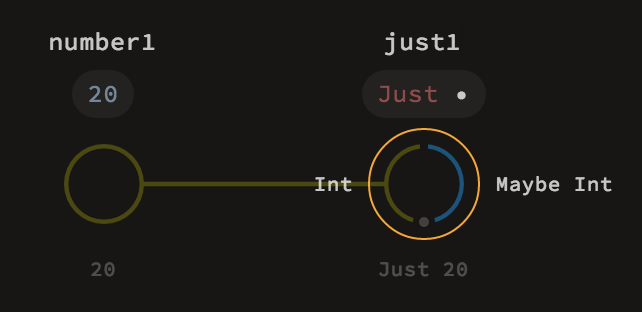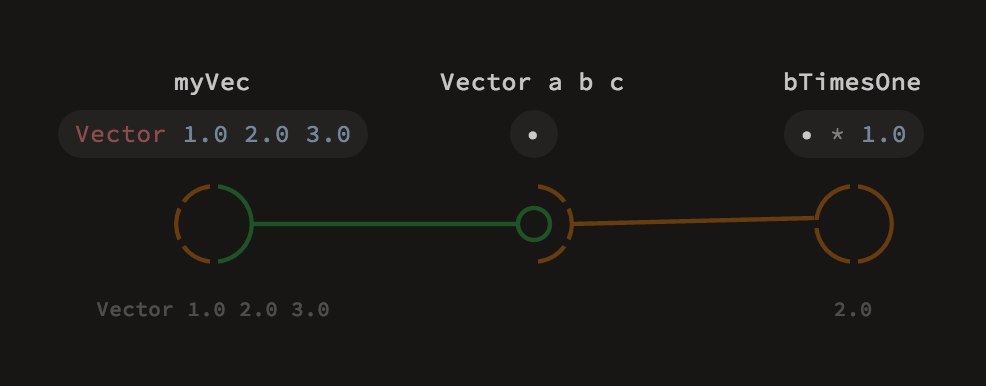# 自定义（数据类型）

## 类和对象

Luna的绑定方法结合面向对象语言已知的途径、纯函数式编程技术，比如代数数据类型（Algebraic Data Type）。

### 简介

Luna中类的概念和大多数已知的编程语言不同。

### 类定义

``````眼下，在可视化编辑器中没法定义类及其方法。本节描述的机制都用来读的，很快就会支持。
``````

``````class Shape:
Circle:
radius :: Real
Rectangle:
width :: Real
height :: Real

def perimeter: case self of
Circle r: 2.0 * pi * r
Rectangle w h: 2.0 * w + 2.0 * h

def area: case self of
Circle r: pi * r * r
Rectangle w h: w * h
``````

### 构造器

``````class Shape:
Circle:
center :: Point
radius :: Real
Rectangle:
topLeft :: Point
bottomRight :: Point
``````

``````class Shape:
Circle Point Real
Rectangle Point Point
``````

``````目前，当只给出一个明确的构造器时，Luna允许通过字段名访问之。该行为很快会扩展来兼容多个构造器的类。
``````

``````class Vector:
x y z :: Real
``````

## 构造器和模式匹配

• 提供创建给定类对象的一种途径。
• 当作模式用来判定使用哪个构造器创建的泪对象并访问其字段。

### 对象构造

``````class Maybe a:
Just a
Nothing
``````

``````Just :: a -> Maybe a
Nothing :: Maybe a
``````### 模式匹配

``````class Vector:
x y z :: Real

myVec = Vector 1.0 2.0 3.0
Vector a b c = myVec
bTimeOne = b * 1.0
``````### Case表达式

``````def render shape:
case shape of
Circle c r: drawCircle c r
Rectangle tl br: drawRectangle tl br
``````

• 在“case...of”之间需要提供需要判定身份的对象。
• 提供一系列从句，每条返回值用于特定构造器场景。

## 多态机制

### 类型参数

Luna类也可以提供类型参数，某些值让自身多态。

``````class Vector:
x y z :: Real
``````

``````class Vector a:
x y z :: a
``````

``````Vector "hello" "world" "!" :: Vector Text
Vector 1 2 3 :: Vector Int
Vector 1.0 2.0 3.0 :: Vector Real
``````### Constrained方法

``````class Vector a:
x y z :: a
def dotProduct that:
self.x * that.x + self.y * that.y + self.z * that.z
``````

``````Vector 1 2 3 . dotProduct (Vector 4 5 6) # 返回32::Int
Vector 1.0 2.0 3.0 . dotProduct (Vector 4.0 5.0 6.0) # 返回32.0::Real
Vector "hello" "world" "!" . dotProduct (Vector "foo" "bar" "baz") # 不会编译
``````